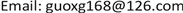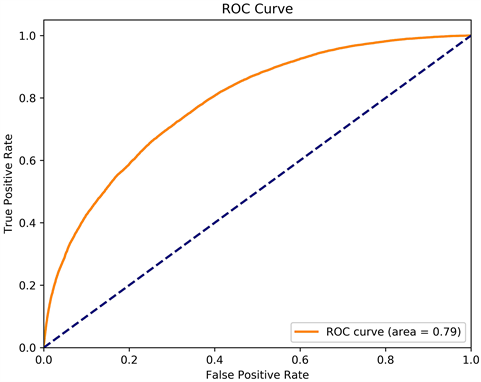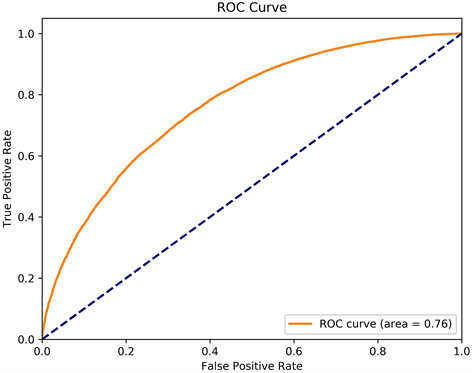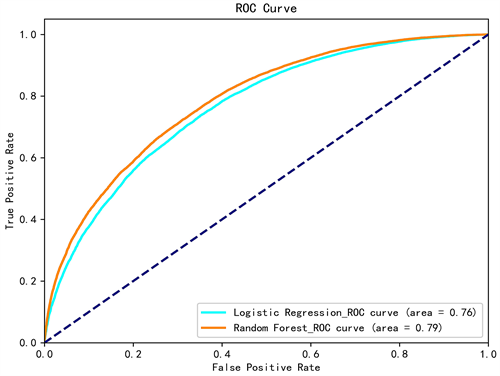﻿ 基于随机森林与逻辑回归模型的交通事故严重程度的预测研究 Prediction of Traffic Accident Severity Based on Random Forest and Logistic Regression Model

Computer Science and Application
Vol. 09  No. 10 ( 2019 ), Article ID: 32641 , 8 pages
10.12677/CSA.2019.910215

Prediction of Traffic Accident Severity Based on Random Forest and Logistic Regression Model

Xiaogang Guo1, Tong Li2

1School of Software, Yunnan University, Kunming Yunnan

2School of Big Data, Yunnan Agricultural University, Kunming YunnanReceived: Oct. 2nd, 2019; accepted: Oct. 17th, 2019; published: Oct. 24th, 2019ABSTRACT

Traffic safety is closely related to people’s lives. The severity of traffic accidents has a great impact on society and people’s lives. This paper chooses random forest and logistic regression algorithm to construct a traffic accident severity prediction model, and makes a prediction and comparative analysis of the severity of traffic accidents. It shows that stochastic forest model has better prediction effect, and ranks the characteristics that affect the severity of traffic accidents. It can judge which factors have greater impact on the severity of traffic accidents. It provides reference and suggestions for traffic road infrastructure construction, as well as for the prevention and reduction of the severity of traffic accidents.

Keywords:Traffic Safety, Traffic Accident Severity, Random Forest, Logistic Regression

1云南大学软件学院，云南 昆明

2云南农业大学大数据学院，云南 昆明1. 引言

2. 数据描述与相关变量说明

2.1. 数据的描述

2.2. 交通事故严重程度的划分

2.3. 部分变量说明

3. 模型的建立与实验结果分析

3.1. 模型的建立

3.1.1. 随机森林模型

(1) 对于 $m=1,2,\cdots ,M:$

(a) 随机的从原始样本集中抽取t个样本点，得到一个训练集 ${A}_{m}$

(b) 使用得到的训练集 ${A}_{m}$ 训练一个CART决策树，在训练时每个节点的切分是在所有特征中随机选择K个特征，然后在这K个特征中选择最佳分割点来划分左右子树。

(2) 对于分类算法中，最终预测类别是样本点所属叶节点处投票最多的类别；对于回归算法，最终类别是样本点所属的叶节点的平均值。

(1) 在处理数据量较大的样本时高度并行化，训练速度较快。

(2) 随机森林运用集成算法，因此准确性比单个算法高。

(3) 对训练样本有很好的适应能力，可以处理维度较高的数据，也可以处理离散型和连续型数据。

(4) 随机森林使用了随机采样，可以明显改善决策树过拟合问题。

3.1.2. 逻辑回归模型

(1) 首先需要找一个合适的预测函数，表示为h函数，该函数是我们要找的分类函数，也是预测输入数据的判断结果 。利用Sigmoid函数和线性回归函数可以得出h函数。

Sigmoid函数：

$g\left(z\right)=\frac{1}{1+{\text{e}}^{-z}}$ (1)

$z={\theta }_{0}+{\theta }_{1}{x}_{1}+{\theta }_{2}{x}_{2}+\cdots +{\theta }_{i}{x}_{i}={\theta }^{\text{T}}x$ (2)

${h}_{\theta }\left(x\right)=\frac{1}{1+{\text{e}}^{-{\theta }^{\text{T}}x}}$ (3)

(2) 构建能够描述模型预测值 $h\left(\theta \right)$ 与真实值y之间的偏差函数 $C\left(\theta \right)$，称为代价函数。代价函数求平均值记作 ，一个模型的优劣可以通过 $B\left(\theta \right)$ 来行判断，当 $B\left(\theta \right)$ 函数越小，表明当前模型与参数更适合训练样本。基于最大似然估计可以达到 $B\left(\theta \right)$

$B\left(\theta \right)=-\frac{1}{m}\left[\underset{i=1}{\overset{m}{\sum }}\left({y}^{\left(i\right)}\mathrm{log}{h}_{\theta }\left({x}^{\left(i\right)}\right)\right)+\left(1-{y}^{\left(i\right)}\right)\mathrm{log}\left(1-{h}_{\theta }\left({x}^{\left(i\right)}\right)\right)\right]$ (4)

(3) 求 $B\left(\theta \right)$ 的最小值使用梯度下降法，使用梯度下降法来求最小值是常用的方法。各个参数的偏导数就是 $B\left(\theta \right)$ 的梯度，机器学习的过程中参数下降的方向就是偏导数的方向，学习率用 $\lambda$ 表示，通过偏导数使用梯度下降法求出使 $B\left(\theta \right)$ 最小的 $\theta$，在对参数进行更新。推导后可得出 ${\theta }_{j}$ 如下：

${\theta }_{j}={\theta }_{j}-\lambda \left(\frac{1}{m}\right)\underset{i=1}{\overset{m}{\sum }}\left({h}_{\theta }\left({x}^{\left(i\right)}\right)-{y}^{\left(i\right)}\right){x}_{j}^{\left(i\right)}$ (5)

3.2. 度量指标

(1) 准确率

$\text{acc}=\frac{1}{n}\underset{i=1}{\overset{n}{\sum }}I\left(f\left({x}_{i}\right)={y}_{i}\right)$ (6)

(2) AUC是ROC曲线下的面积，AUC的值是一个概率值，当AUC的值越大，则表示当前的分类模型拥有更好的分类能力。

(3) F1-measure是查准率和查全率的加权调和平均数，对于一些非平衡的数据集，难以估计小类样本对预测结果的影响，因此通过查准率和查全率能够够有很好的评价。F1-measure计算公式如下：

$\text{F}1=\frac{2PR}{P+R}$ (7)

3.3. 实验结果与对比分析

3.3.1. 实验结果Figure 1. Random forest model ROC curveFigure 2. Logistic regression model ROC curve

3.3.2. 对比分析Figure 3. Comparison between random forest and logistic regression model ROC curve

(1) 随机森林模型的预测准确率为0.75，逻辑回归模型的预测准确率为0.71，可见随机森林模型预测效果更好，优于逻辑回归模型。

(2) 从F1-measure值看，随机森林模型F1-measure值为0.72，逻辑回归模型F1-measure为0.70，可见随机森林模型进行预测时比起逻辑回归模型更加稳定。

(3) 从图3中可以得知随机森林模型AUC的值比逻辑回归模型的值要高一些，随机森林模型分类效果要比逻辑回归模型表现更好。

4. 总结

(1) 本文构建的两个交通事故严重程度预测模型都能够预测交通事故的严重程度，其次交通事故数据具有很多的维度，随机森林算法对于多维数据有很好的适应能力，并且能够获得较好的预测效果。

(2) 通过对交通事故的严重程度进行预测，在研究的过程中知道是否超速，天气状况、事故发生的时间和地点、光照条件等因素都与交通事故严重程度有较大的关系。因此在交通事故严重程度进行预防或降低交通事故严重程度时都有很好的借鉴作用，在建设交通道路时可以尽量考虑这些因素，规划到设计之中，从而能够降低对交通事故造成的影响。

(3) 本文只使用了随机森林与逻辑回归两种建模算法，后续可以研究其它算法来进行验证，以期能够得到更好的预测效果。

Prediction of Traffic Accident Severity Based on Random Forest and Logistic Regression Model[J]. 计算机科学与应用, 2019, 09(10): 1920-1927. https://doi.org/10.12677/CSA.2019.910215

1. 1. Ahmed, M.M. and Abdel-Aty, M.A. (2012) The Viability of Using Automatic Vehicle Identification Data for Real-Time Crash Prediction. IEEE Transactions on Intelligent Transportation Systems, 13, 459-468. https://doi.org/10.1109/TITS.2011.2171052

2. 2. Shinohara, K., Okazaki, J., Sakuma, H., et al. (2003) A Clinical Survey of Motor Vehicle Crashes: What Most Influences the Severity of Patient’s Injuries? JSAE Review, 24, 357-358. https://doi.org/10.1016/S0389-4304(03)00040-7

3. 3. Olutayo, V.A. and Eludire, A.A. (2014) Traffic Accident Analysis Using Decision Trees and Neural Networks. International Journal of Information Technology and Computer Science, 6, 22-28. https://doi.org/10.5815/ijitcs.2014.02.03

4. 4. 李庚凭. 基于有序Logit和多项Logit模型的高速公路交通事故严重程度预测[D]: [硕士学位论文]. 西安: 长安大学, 2018.

5. 5. Breiman, L. (2001) Random For-ests. Machine Learning, 45, 5-32. https://doi.org/10.1023/A:1010933404324

6. 6. Breiman, L. (1996) Bagging Predictors. Machine Learning, 24, 123-140. https://doi.org/10.1007/BF00058655

7. 7. Menezes, F.S.D., Liska, G.R., Cirillo, M.A., et al. (2017) Data Classification with Binary Response through the Boosting Algorithm and Logistic Regression. Expert Systems with Applications, 69, 62-73. https://doi.org/10.1016/j.eswa.2016.08.014

8. 8. Lu, T., Zhu, D., Yan, L., et al. (2015) The Traffic Accident Hotspot Prediction: Based on the Logistic Regression Method. International Conference on Transportation Information & Safety, Wuhan, 25-28 June 2015, 107-110. https://doi.org/10.1109/ICTIS.2015.7232194

9. 9. 李卓冉. 逻辑回归方法原理与应用[J]. 中国战略新兴产业, 2017(28): 125-126.